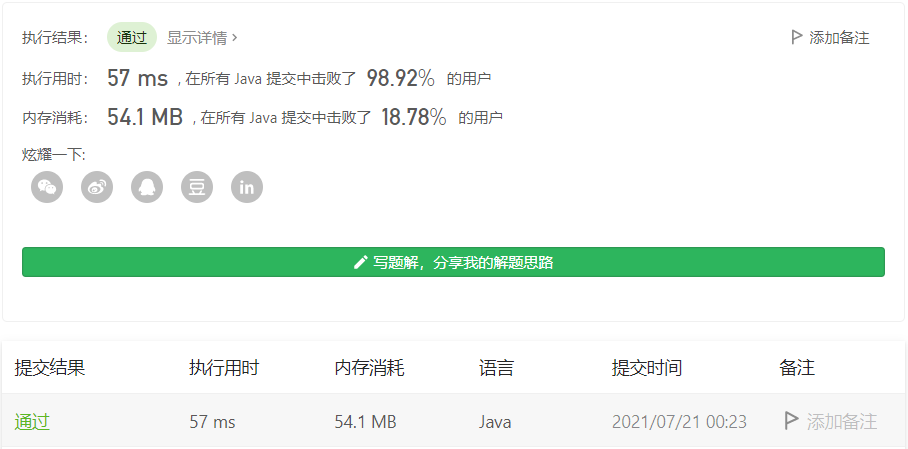# 1877. 数组中最大数对和的最小值

#### 2021-07-21 LeetCode每日一题

• 比方说，如果我们有数对 (1,5) ，(2,3) 和 (4,4)，最大数对和 为 max(1+5, 2+3, 4+4) = max(6, 5, 8) = 8 。

• nums 中每个元素 恰好 在 一个 数对中，且
• 最大数对和 的值 最小 。

输入：nums = [3,5,2,3]



输入：nums = [3,5,4,2,4,6]



• n == nums.length
• 2 <= n <= 105
• n 是 偶数 。
• 1 <= nums[i] <= 105

class Solution {
public int minPairSum(int[] nums) {
Arrays.sort(nums);
int left = 0, right = nums.length - 1;
int res = Integer.MIN_VALUE;

while (left < right) {
int sum = nums[left] + nums[right];
if (sum > res) {
res = sum;
}
left++;
right--;
}

return res;
}
}posted @ 2021-07-21 00:32  CodeTiger  阅读(13)  评论(0编辑  收藏  举报